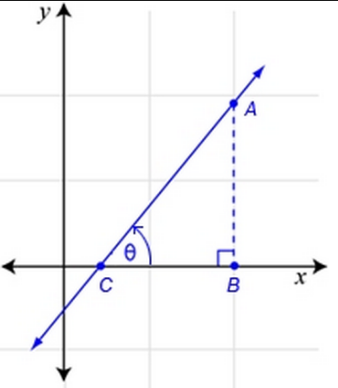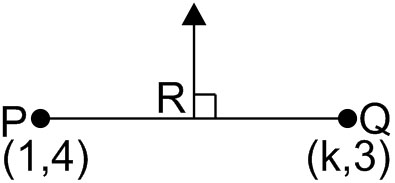Q

# Can someone explain - Co-ordinate geometry - JEE Main-4

The perpendicular bisector of the line segment joining has intercept – 4 . Then a possible value of is

• Option 1)

– 4

• Option 2)

1

• Option 3)

2

• Option 4)

– 2

123 Views

As we learnt in

Slope of a line -

If  is the angle at which a straight line is inclined to a positive direction of x-axis, then the slope is defined by

.

- whereinand,

Mid-point formula -

- wherein

If the point P(x,y) is the mid point of line joining A(x1,y1) and B(x2,y2) .

As well as,

Slope – point from of a straight line -

- wherein

slope

point through which line passesMid-point of     is

Slope of        is

Slope of line perpendicular to

so point is

Option 1)

– 4

This option is correct.

Option 2)

1

This option is incorrect.

Option 3)

2

This option is incorrect.

Option 4)

– 2

This option is incorrect.

Exams
Articles
Questions